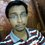# Problem to give solutions of the weekly challenged problems.

When I post a solution of a weekly challenged problem, I can not see my solution in the proper view. I post the solutions after writing them in 'LATEX'. But they come to me in plane text. How can I clarify my fault? Does anybody else also face such a problem or this problem is happening just with me? How should I write the solutions? Can the other users see my solutions in proper view?............. Please help me.....a link of my solution is....https://brilliant.org/assessment/s/algebra-and-number-theory/2937020/ ......you can check one of my written solution in 'LATEX' through this link.

Calvin: Type it as such $$\frac{a}{b}$$.Note by Debjit Mandal
5 years, 8 months ago

MarkdownAppears as
*italics* or _italics_ italics
**bold** or __bold__ bold
- bulleted- list
• bulleted
• list
1. numbered2. list
1. numbered
2. list
Note: you must add a full line of space before and after lists for them to show up correctly
paragraph 1paragraph 2

paragraph 1

paragraph 2

[example link](https://brilliant.org)example link
> This is a quote
This is a quote
    # I indented these lines
# 4 spaces, and now they show
# up as a code block.

print "hello world"
# I indented these lines
# 4 spaces, and now they show
# up as a code block.

print "hello world"
MathAppears as
Remember to wrap math in $$...$$ or $...$ to ensure proper formatting.
2 \times 3 $$2 \times 3$$
2^{34} $$2^{34}$$
a_{i-1} $$a_{i-1}$$
\frac{2}{3} $$\frac{2}{3}$$
\sqrt{2} $$\sqrt{2}$$
\sum_{i=1}^3 $$\sum_{i=1}^3$$
\sin \theta $$\sin \theta$$
\boxed{123} $$\boxed{123}$$

Sort by:

$$2+3$$

- 4 years, 11 months ago

How can I write a fraction in latex? Is it \frac {a}{b} ? But, this format does not show the fraction a/b. I got this text from the Formatting guide! What is the right format?......please help me.

Calvin: Type it as such $$\frac{a}{b}$$.

- 5 years, 8 months ago

As several others have mentioned, you need to place your Latex code within \ ( \ ) (with the spaces removed. So, instead of \frac{a}{b}, it will appear as $$\frac{ a} {b}$$.

I'd add a line to your comment and your post, so that you can view how to write it properly, by choosing the edit option to see what I typed.

Staff - 5 years, 8 months ago

Thank you very much. I used \ ( \ ) (with the spaces removed) like ''$$frac{a}{b}$$'' but not like $$\frac{a}{b}$$. I shall try this in the solutions. THANKS.

- 5 years, 8 months ago

oh! Very very thanks. I got it. That helped me so much, Mursalin.

- 5 years, 8 months ago

You're welcome!

- 5 years, 8 months ago

Yeah, I submit solutions through that 'Formatting guide'. That 'Formatting guide' is just a guide to write in 'LATEX'. I always follow that. Can you please go through the above link of my solution, to check if there is any problem of my solution writing? I am not understanding why this thing is happening!

- 5 years, 8 months ago

That link's not going to work for everyone (for instance: me). Try copying your solution and paste it as a comment on this thread.

- 5 years, 8 months ago

Recurrence Relation a{n} - a{n-1}=n(a{n-1} - a{n-2}), a{n-1} - a{n-2}=(n-1)(a{n-2} - a{n-3}), a{n-2} - a{n-3}=(n-2)(a{n-3} - a{n-4}), . . a{3} - a{2}=3(a{2} - a{1})=3(3-1)=3*2, a{2} - a{1}=3-1=2; So, we can easily find that, a{n} - a{n-1} = n!, a{n-1} - a{n-2}=(n-1)!, a{n-2} - a{n-3}=(n-2)!, . . a{3} - a{2}=3!, a{2} - a{1}=2!; By adding these (n-1) equations, all the a{i} 's will be canceled out, where i is a positive integer and 2 \leq i \leq (n-1). So, we shall get, a{n} - a{1} = \displaystyle \sum{j=2}^n j! \Rightarrow a{n}= \displaystyle \sum{j=1}^n j! [where a{1}=1, given]. Note that, 11 divides \displaystyle \sum{j=1}^10 j! and for all positive integer k \geq 11; 11 also divides k!. So, when n \geq 10, a{n} is divisible 11. So, for 10 \leq n \leq 1000, there are 991 such a{n}'s. Now we just need to find, how many a{n}'s are divisible by 11, for 1 \leq n \leq 9. It is very easy to calculate a{n}, where 1 \leq n \leq 9. Then, we shall find that only a{4} and a{8} are divisible by 11. So, the total number of such a_{n} is (991+2) = 993 [answer]

- 5 years, 8 months ago

Oh! You forgot to wrap your solution inside the "slash brackets". Try typing \ (2+3\ ) without the white-spaces in between the \ & the brackets. Then click on preview. You should see something like this:

$$2+3$$.

Hope this helps!

- 5 years, 8 months ago

It's a very simple problem. You have just forgotten to put  around your latex.

- 5 years, 8 months ago

Did you check out the Formatting guide, which is to the left of the Preview and Post buttons?

- 5 years, 8 months ago